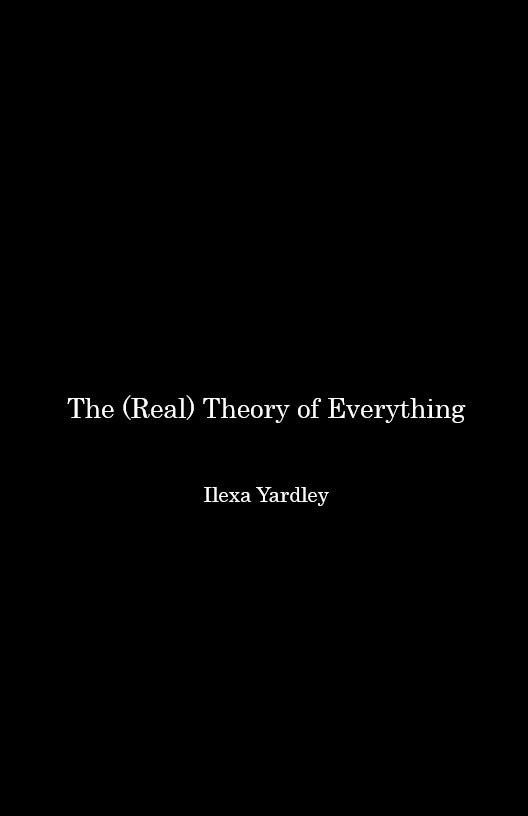# Standardizing the Hydrogen Wave (Function)

Source: Deep Learning on Medium# Standardizing the Hydrogen Wave (Function)

Zero equals one (two, three, four).

Normally, we ‘think’ of the ‘hydrogenatom as one proton and one electron.

Because zero and one is circumference and diameter. Separated. And, joined (superimposed).

This explains why we need (why we use) three positions (states) to articulate the hydrogen wave (function).

Allowing us to ‘remove’ (understand) (articulate) the ‘duplicity’ (redundancy) required to articulate (symbolize) anything.

Therefore, a better way to articulate any atom (any unit) is (0 (1) 0) .

Explaining, if you want to go there, a human ‘face.’ (Any face.) The required circular relationship between an observer and an observation.

Therefore, to go back to the hydrogen atom (wave function) (2,0,0) (3,0,0) (2,1,0) (3,1,0) (3,1,1) (2,1,1) (3,2,0) (3,2,1) (3,2,2) (4,0,0) (4,1,0) (4,1,1) (4,2,0) (4,2,1) (4,2,2) (4,3,0) (4,3,1) (4,3,2) (4,3,3) are different ways to articulate the same reality (different state is same state).

Meaning, more technically, zero equals one.

Because, from pi’s point of view, circumference is diameter (any zero and-or one).

Because conservation of the circle is the core (only) dynamic in Nature.

Invest in the Circular Theory…